# Subseries convergence

(diff) ← Older revision | Latest revision (diff) | Newer revision → (diff)
If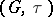is a Hausdorff Abelian topological group, a series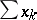inis-subseries convergent (respectively, unconditionally convergent) if for each subsequence(respectively, each permutation) of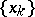, the subseries(respectively, the rearrangement) is-convergent in. In one of the early papers in the history of functional analysis, W. Orlicz showed that ifis a weakly sequentially complete Banach space, then a series inis weakly unconditionally convergent if and only if the series is norm unconditionally convergent [a5]. Later, he noted that if "unconditional convergence" is replaced by "subseries convergence" , the proof showed that the weak sequential completeness assumption could be dropped. That is, a series in a Banach space is weakly subseries convergent if and only if the series is norm subseries convergent; this result was announced in [a1], but no proof was given. In treating some problems in vector-valued measure and integration theory, B.J. Pettis needed to use this result but noted that no proof was supplied and then proceeded to give a proof ([a6]; the proof is very similar to that of Orlicz). The result subsequently came to be known as the Orlicz–Pettis theorem (see [a3] for a historical discussion).
In the case of a Banach space, attempts have been made to replace the weak topology ofby a weaker topology,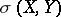, generated by a subspaceof the dual space ofwhich separates the points of. Perhaps the best result in this direction is the Diestel–Faires theorem, which states that ifcontains no subspace isomorphic to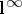, then a series inissubseries convergent if and only if the series is norm subseries convergent. Ifis the dual of a Banach space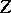and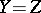, then the converse also holds (see [a2], for references and further results).